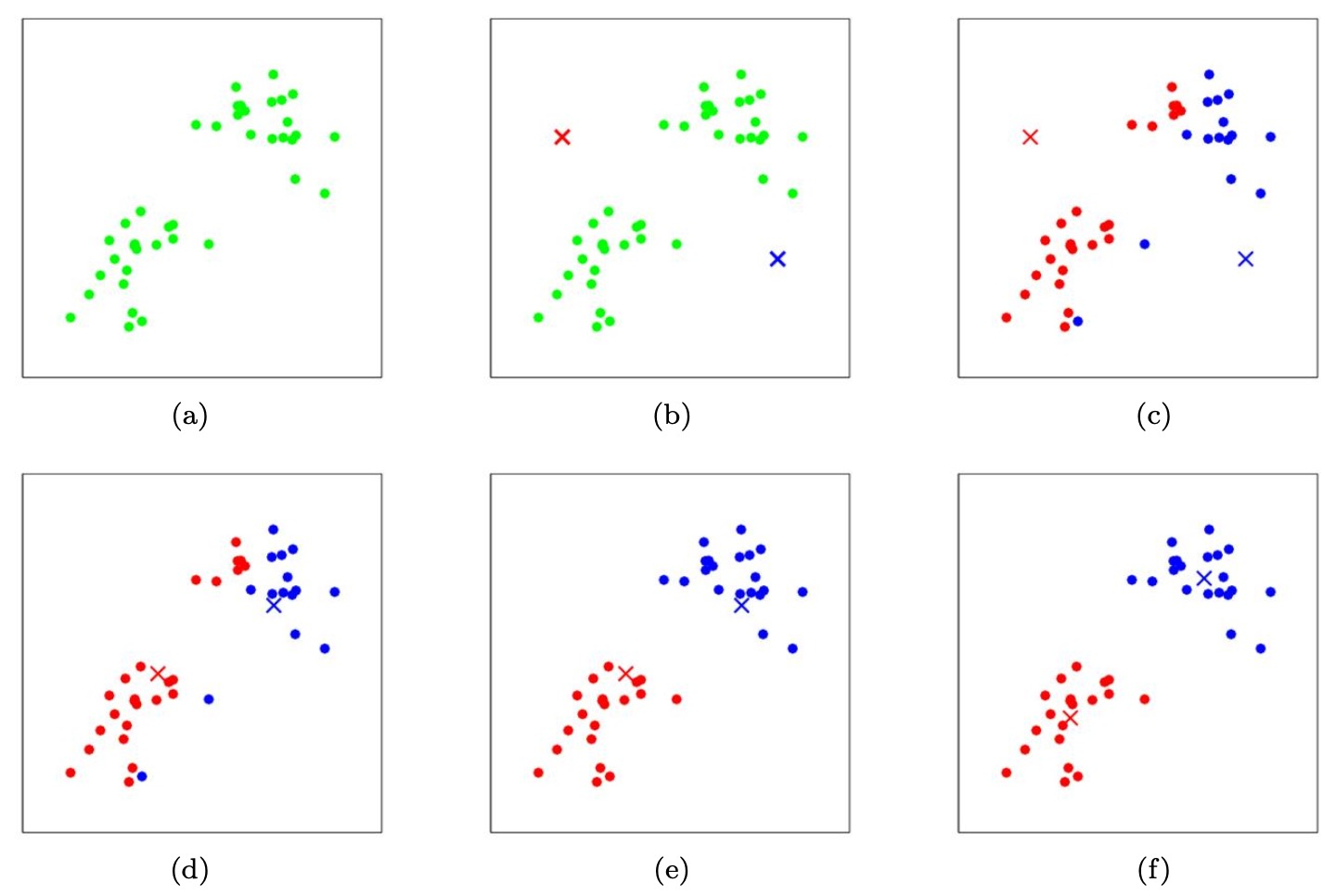## The $$k$$-means clustering algorithm

• In the clustering problem, we are given a training set $$\left\{x^{(1)}, \ldots, x^{(m)}\right\}$$, and want to group the data into a few cohesive “clusters.” Here, $$x^{(i)} \in \mathbb{R}^{n}$$ as usual; but no labels $$y^{(i)}$$ are given. So, this is an unsupervised learning problem. The $$k$$-means clustering algorithm is as follows:

1. Initialize cluster centroids $$\mu_{1}, \mu_{2}, \ldots, \mu_{k} \in \mathbb{R}^{n}$$ randomly.
2. Repeat until convergence:

• For every $$i$$, set,
$c^{(i)}:=\operatorname*{arg\,min}_{j}\left\|x^{(i)}-\mu_{j}\right\|^{2}$
• For each $$j$$, set,
$\mu_j:=\frac{\sum_{i=1}^{m} \mathbb{1}\{c^{(i)}=j\} x^{(i)}}{\sum_{i=1}^{m} \mathbb{1}\{c^{(i)}=j\}}$
• where $$\mathbb{1}\{\cdot\}$$ represents the indicator function which returns 1 if its argument is true, otherwise 0. In other words, the indicator of a true statement is equal to 1 while that of a false statement is equal to 0.
• In the algorithm above, $$k$$ (a parameter of the algorithm) is the number of clusters we want to find; and the cluster centroids $$\mu_{j}$$ represent our current guesses for the positions of the centers of the clusters. To initialize the cluster centroids (in step 1 of the algorithm above), we could choose $$k$$ training examples randomly, and set the cluster centroids to be equal to the values of these $$k$$ examples. (Other initialization methods are also possible.)
• The inner-loop of the algorithm repeatedly carries out two steps:
• “Assigning” each training example $$x^{(i)}$$ to the closest cluster centroid $$\mu_{j}$$ and,
• Moving each cluster centroid $$\mu_{j}$$ to the mean of the points assigned to it. Figure 1 shows an illustration of running $$k$$-means.
• The figure below shows the K-means algorithm in action. Training examples are shown as dots, and cluster centroids are shown as crosses.
• (a) Original dataset.
• (b) Random initial cluster centroids (in this instance, not chosen to be equal to two training examples).
• (c-f) Illustration of running two iterations of $$k$$-means. In each iteration, we assign each training example to the closest cluster centroid (shown by “painting” the training examples the same color as the cluster centroid to which is assigned); then we move each cluster centroid to the mean of the points assigned to it.
•  Images courtesy [CS281A/Stat241A: Statistical Learning Theory K-means and Expectation Maximization by Michael Jordan, UC Berkeley](http://people.eecs.berkeley.edu/~jordan/courses/281A-fall02/lectures/lecture17.pdf).### Convergence guarantee

• Is the $$k$$-means algorithm guaranteed to converge? Yes it is, in a certain sense. In particular, let us define the distortion function to be:
$J(c, \mu)=\sum_{i=1}^{m}\left\|x^{(i)}-\mu_{c^{(i)}}\right\|^{2}$
• Thus, $$J$$ measures the sum of squared distances between each training example $$x^{(i)}$$ and the cluster centroid $$\mu_{c^{(i)}}$$ to which it has been assigned. It can be shown that $$k$$-means is exactly coordinate descent on $$J$$. Specifically, the inner-loop of $$k$$-means repeatedly minimizes $$J$$ with respect to $$c$$ while holding $$\mu$$ fixed, and then minimizes $$J$$ with respect to $$\mu$$ while holding $$c$$ fixed. Thus, $$J$$ must monotonically decrease, and the value of $$J$$ must converge. Usually, this implies that $$c$$ and $$\mu$$ will converge too.
• In theory, it is possible for $$k$$-means to oscillate between a few different clusterings – i.e., a few different values for $$c$$ and/or $$\mu-$$ that have exactly the same value of $$J$$, but this almost never happens in practice).
• The distortion function $$J(\cdot)$$ is a non-convex function, and so coordinate descent on $$J$$ is not guaranteed to converge to the global minimum. In other words, $$k$$-means can be susceptible to local optima. Very often $$k$$-means will work fine and come up with very good clusterings despite this.
• If you are worried about getting stuck in bad local minima, one common thing to do is run $$k$$-means many times (using different random initial values for the cluster centroids $$\mu_{j}$$). Then, out of all the different clusterings found, pick the one that gives the lowest distortion $$J(c, \mu)$$.

## Citation

If you found our work useful, please cite it as:

@article{Chadha2020DistilledkMeansClusteringAlgorithm,
title   = {k-Means Clustering Algorithm},